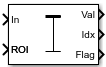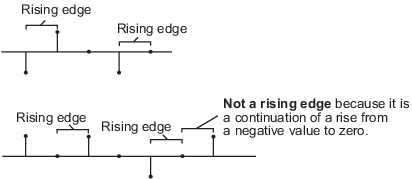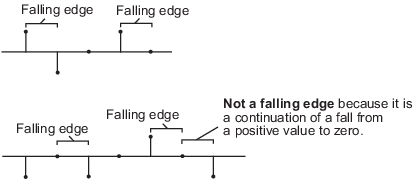# 2-D Maximum

Compute maximum value of input or sequence of inputs

• Library:
• Computer Vision Toolbox / Statistics

•## Description

The 2-D Maximum block identifies the index of the largest element in an input array. The input can be a 1-D vector, 2-D matrix, or an N-D-array. The block can compute the maximum value along a specified dimension of the input or the entire input. The 2-D Maximum block can also track the maximum values in a sequence of inputs over a period of time.

## Ports

### Input

expand all

Input array, specified as a vector, matrix, or N-D- array. This port is unnamed until you select the Enable ROI processing parameter or set the Mode parameter to `Running`.

Data Types: `single` | `double` | `int8` | `int16` | `int32` | `int64` | `uint8` | `uint16` | `uint32` | `uint64` | `Boolean` | `fixed point`
Complex Number Support: Yes

Reset port, specified as a scalar. This port specifies the event that causes the block to reset the running maximum. The sample time of the Rst input must be a positive integer and a multiple of the input sample time.

#### Dependencies

To enable this port, set the Mode parameter to `Running` and set the Reset port parameter to `Rising edge`, ```Falling edge```, `Either edge`, or `Non-zero sample`.

Data Types: `single` | `double` | `int8` | `int16` | `int32` | `uint8` | `uint16` | `uint32` | `Boolean`

Region of interest (ROI), specified as a four-element vector, m-by-4 matrix, or M-by-N matrix. The input value to this port depends on the ROI type parameter.

Note

You can use the `ROI` port only if the input is a matrix.

#### Dependencies

To enable this port, set the Find the maximum value over parameter to `Entire input` and select the Enable ROI processing parameter.

Data Types: `single` | `double` | `int8` | `int16` | `int32` | `uint8` | `uint16` | `uint32`

Label matrix, specified as a matrix of nonnegative integers. The label matrix represents the objects in an image. The pixels labeled 0 are the background. The pixels labeled 1 make up one object; the pixels labeled 2 make up a second object; and so on. The size of the label matrix must be the same size as the 2-D input.

#### Dependencies

To enable this port, select the Enable ROI processing parameter and set the ROI type parameter to `Label matrix`.

Data Types: `uint8` | `uint16` | `uint32`

Label values of ROIs, specified as a M-element vector. The value of M can be less than or equal to the number of objects that are labeled in the label matrix.

#### Dependencies

To enable this port, select the Enable ROI processing parameter and set the ROI type parameter to `Label matrix`.

Data Types: `uint8` | `uint16` | `uint32`

### Output

expand all

Output maximum value, returned as a scalar, vector, matrix, or N-D-array. The size of the returned maximum value depends on the size of the input, and the settings for the Find the maximum value over parameter.

This port is unnamed if Mode is set to `Running`.

#### Compute Maximum Value of Input Array

The Mode parameter is set to ```Value and Index``` or `Value`. In this case, you can compute the maximum value along any specified dimension of the input or the entire input. The output maximum value is a:

• Scalar if the input is of any size and Find the maximum value over parameter is set to ```Entire input```.

• Vector if the input is a matrix and the Find the maximum value over parameter is set to any one of ```Each row```, `Each column`, and `Specified dimension`. In this case, the Dimension value for ```Specified dimension``` can be either 1 or 2.

• (N-1)-D array if the input is an N-D array and the Find the maximum value over parameter is set to `Specified dimension` and the Dimension value is N.

• N-D array with one singleton dimension if the input is an N-D array and the Find the maximum value over parameter is set to either of ```Each row```, `Each column`, and `Specified dimension`.

Example: For a 3-D input array of size M-by-N-by-P, the dimension of the returned output is:

• 1-by-N-by-P if you set the Find the maximum value over parameter to `Entire row`.

• M-by-1-by-P if you set the Find the maximum value over parameter to `Entire column`.

• M-by-N if you set the Find the maximum value over parameter to `Specified dimension` and the Dimension value to 3.

#### Compute Maximum Value of Sequence of Inputs

When you set the Mode parameter to `Running`, the block computes the maximum value relative to each sample in the input sequence. The output is of the same size as the input.

Data Types: `single` | `double` | `int8` | `int16` | `int32` | `uint8` | `uint16` | `uint32` | `fixed point`

Indices of maximum values in the input array, returned as a scalar, vector, matrix, or N-D-array. The size of the returned indices depends on the size of the input, and the settings for the Find the maximum value over parameter.

To enable this port set the Mode parameter to `Value and Index` or `Index`. The indices returned at the output is a:

• Scalar if the input is a N- element vector and Find the maximum value over parameter is set to ```Entire input```.

• Vector if the input is a matrix and the Find the maximum value over parameter is set to any one of ```Entire input```, `Each row`, ```Each column```, and `Specified dimension`. In this case, the Dimension value for `Specified dimension` can be either 1 or 2.

• (N-1)-D array if the input is a N-D array and the Find the maximum value over parameter is set to `Specified dimension` and the Dimension value is N.

• N-D array with one singleton dimension if the input is an N-D array. In this case the Find the maximum value over parameter is set to ```Each row```, `Each column`, or `Specified dimension`. In this case, the Dimension value for ```Specified dimension``` is an integer less than N.

Example: For a 3-D input array of size M-by-N-by-P, the dimension of the returned output is:

• 1-by-N-by-P if you set the Find the maximum value over parameter to `Entire row`.

• M-by-1-by-P if you set the Find the maximum value over parameter to `Entire column`.

• M-by-N if you set the Find the maximum value over parameter to `Specified dimension` and the Dimension value to 3.

Note

When a maximum value occurs more than once, the computed index corresponds to the first occurrence. For example, in the input vector ```[3 2 1 2 3]```, the maximum value is 3 and the one-based index of the maximum value is `1`.

Data Types: `single` | `double` | `int8` | `int16` | `int32` | `int64` | `uint8` | `uint16` | `uint32` | `uint64` | `Boolean` | `fixed point` | `enumerated` | `bus`

Validation for ROI, returned as 0 or 1. The output value signifies if all of the ROIs specified at the input lie within the image bounds. The output value depends on the values of the Output and ROI Type parameters.

 ROI Type Output Output from Flag port Description `Rectangles``Lines` ```Individual statistics for each ROI``` 0 ROI is completely outside the input image. 1 ROI is either completely or partially inside the input image. ```Single statistic for all ROIs``` 0 ROI is completely outside the input image. 1 ROI is either completely or partially inside the input image. `Label matrix` ```Individual statistics for each ROI``` 0 Label number is not in the label matrix. 1 Label number is in the label matrix. ```Single statistic for all ROIs``` 0 None of the label numbers are in the label matrix. 1 At least one of the label numbers is in the label matrix.

Note

If the ROI is partially outside the image, the block computes the variance values only for the portion of the ROI that lies within the image bounds.

#### Dependencies

To enable this port, select the Output flag indicating if ROI is within image bounds parameter and set the value of ROI type parameter to `Rectangle`, `Lines`, or ```Label Matrix```.

## Parameters

expand all

Main Tab

Specify the type of output to be returned by the block. Select the output mode as:

• `Value and Index` to return the maximum values and the corresponding indices in the given input.

• `Value` to return only the computed maximum values.

• `Index` to return only the indices of the maximum values in the given input.

• `Running` to return the maximum values in a sequence of inputs.

Specify the index for the first element in the input array. Select the index origin as:

• `One` for one-based numbering. The range of the index values for the elements in an N-D input array is set to (1, N). For example, the index value for the first element in the matrix is set to (1,1).

• `Zero` for zero-based numbering. The range of the index value for the elements in an N-D input array is set to (0, N-1). For example, the index value for the first element in the matrix is set to (0,0).

Specify the dimension of the input along which the block computes the maximum.

• `Entire input` — Computes maximum over the entire input.

• `Each row` — Computes maximum over each row.

• `Each column` — Computes maximum over each column.

• `Specified dimension`— Computes maximum over the dimension specified in the Dimension parameter.

• If Dimension is 1, the output is the same as when you select `Each column`.

• If Dimension is 2, the output is the same as when you select `Each row`.

#### Dependencies

To enable this parameter, set the Mode parameter to `Value and Index`, `Value`, or `Index`.

Specify the dimension of the input array over which the maximum is computed as a one-based value. The value of this parameter must be greater than 0 and less than or equal to the number of dimensions in the input array.

#### Dependencies

To enable this parameter, set the Find the maximum value over parameter to `Specified dimension`.

Specify when the block detects a reset event. The block resets the running mode when a reset event is detected at the optional Rst port. The reset sample time must be a positive integer and a multiple of the input sample time.

Specify the reset event as:

• `None` to disable the Rst port.

• `Rising edge` to trigger a reset event when the Rst input does one of the following:

• Rises from a negative value to either a positive value or zero

• Rises from zero to a positive value, where the rise is not a continuation of a rise from a negative value to zero• `Falling edge` to trigger a reset event when the Rst input does one of the following:

• Falls from a positive value to either a negative value or zero

• Falls from zero to a negative value, where the fall is not a continuation of a fall from a positive value to zero• `Either edge` to trigger a reset event when the Rst input is a ```Rising edge``` or `Falling edge`.

• `Non-zero sample` to trigger a reset event at each sample time, when the Rst input is not zero.

Note

When running simulations in the Simulink® multitasking mode, reset signals have a one-sample latency. Therefore, when the block detects a reset event, there is a one-sample delay at the reset port rate before the block applies the reset.

#### Dependencies

To enable this parameter, set the Mode parameter to `Running`.

ROI Processing

Select to calculate maximum within a particular ROI in the image.

Note

Full ROI processing is available only if you have a Computer Vision Toolbox™ license. If you do not have a Computer Vision Toolbox license, you can still use ROI processing, but the ROI type is limited to `Rectangles`.

#### Dependencies

To enable this parameter, set the Find the maximum value over parameter to `Entire input`.

Specify the type of ROI that represents the regions in the image over which the block computes the variance. The type of ROI can be a rectangle, line, label matrix, or a binary mask.

 Parameters Description ROI type Inputs to the ROI port `Rectangles` Four-element row vector ```[x y width height]```m-by-4 matrix `$\left[\begin{array}{cccc}{x}_{1}& {y}_{1}& widt{h}_{1}& heigh{t}_{1}\\ {x}_{2}& {y}_{2}& widt{h}_{2}& heigh{t}_{2}\\ ⋮& ⋮& ⋮& ⋮\\ {x}_{M}& {y}_{M}& widt{h}_{M}& heigh{t}_{M}\end{array}\right]$`,where m is the number of rectangles. Each row of the matrix corresponds to a different rectangle. `x` and `y` are the one-based coordinates of the upper left corner of the rectangle.`width` and `height` are the width and height, in pixels, of the rectangle. The values of `width` and `height` must be greater than 0. `Lines` Four-element row vector ```[x1 y1 x2 y2]```m-by-4 matrix `$\left[\begin{array}{cccc}{x}_{11}& {y}_{11}& {x}_{12}& {y}_{12}\\ {x}_{21}& {y}_{21}& {x}_{22}& {y}_{22}\\ ⋮& ⋮& ⋮& ⋮\\ {x}_{M1}& {y}_{M1}& {x}_{M2}& {y}_{M2}\end{array}\right]$`,where m is the number of lines. Each row of the matrix corresponds to a different line. `x1` and `y1` are the coordinates of the beginning of the line.`x2` and `y2` are the coordinates of the end of the line. `Label matrix` M-by-N matrix Matrix of the same size as the input image. The matrix contains label values that represent different objects in an image. The pixels labeled 0 are the background. The pixels labeled 1 make up one object; the pixels labeled 2 make up a second object; and so on. `Binary mask` M-by-N matrix Matrix of the same size as the input image. The binary mask classifies image pixels as belonging to either the region of interest or the background. The mask pixel values of 1 indicate that the image pixel belongs to the ROI. The mask pixel values of 0 indicate that the image pixel is part of the background.

#### Dependencies

To enable this parameter, set the Find the maximum value over parameter to `Entire input` and select the Enable ROI processing parameter.

Specify the portion of the ROI from which the block has to calculate 2-D maximum. The ROI portion is either the entire ROI or the ROI perimeter.

#### Dependencies

To enable this parameter, select the Enable ROI processing parameter and set the ROI type parameter to `Rectangles`.

Specify whether to calculate 2-D maximum individually for each ROI or for the entire ROI.

• If you select `Individual statistics for each ROI`, the block outputs a vector of maximum values. The size of the output vector is equal to the number of ROIs.

• If you select `Single statistic for all ROIs`, the block outputs a scalar value. The scalar value represents the statistical value for all the specified ROIs.

#### Dependencies

To enable this parameter, select the Enable ROI processing parameter and set the ROI type parameter to `Rectangles`, `Lines`, or `Label matrix`.

Select to expose the Flag port. For a description of the Flag port output, see Flag.

#### Dependencies

To enable this parameter, select the Enable ROI processing parameter and set the ROI type parameter to `Rectangles`, `Lines`, or `Label matrix`.

Data Types Tab

For details on the fixed-point block parameters, see Specify Fixed-Point Attributes for Blocks.

Select this parameter to prevent the fixed-point tools from overriding the data types you specify in this block. For more information, see Lock the Output Data Type Setting (Fixed-Point Designer).

## Block Characteristics

 Data Types `double` | `fixed point` | `integer` | `single` Multidimensional Signals `no` Variable-Size Signals `yes`

expand all